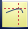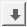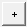Demand Model in Excel
The formula in cell B8 is a calculation of the Overall Cost associated with having different amounts of product in stock. The formula, which is shown in the Formula Bar, is a function of four cells:
 • Amount Stocked is the amount of product in stock.
 • Demand is the customer demand for the product.
 • Air Freight is the cost per unit to ship additional product by air when the demand exceeds the amount in stock.
 • Expiration Cost is the cost per unit of disposing of unused product when the demand is less than the amount in stock.
 • If Amount Stocked is less than Demand, then the company has to ship additional units, at a cost of (Demand-Amount Stocked) × Air Freight. For example, if the demand is 8, but the company has only 6 in stock, then it has to ship 8 - 6 = 2 units at a cost of 2 × 150 = 300.
 • If Amount Stocked is greater than Demand, then the company has to dispose of unused product, at a cost of (Amount Stocked - Demand) × Expiration Cost. For example, if the demand is 5, but the company has 8 in stock, then it has to dispose of 8 - 5 = 3 units at a cost of 3 × 50 = 150.
 • If Amount Stocked is equal to Demand, then there is no shipping cost or disposal cost.
 • There is never both a shipping cost and a disposal cost at the same time.
 1 Select Help > Sample Data Library and navigate up one level to the Sample Import Data folder.
 2 Double-click Demand.xls to open the file in Microsoft Excel.
 3 In Microsoft Excel, click the JMP ribbon.
 4 Click the Create/Edit Model button.
 5
 6 Select Air Freight in the Inputs box and then click the down arrow button.
 7 Click OK.
Notes:
 • If the fields in the Create/Edit Model window are not populated when you set up your model, click Choose and select the cell that contains the input name. Specify the values and click Apply.
 • You can create more than one model for a worksheet. In the Create/Edit Model window, click the plus buttonnext to the Model name. In the Model Name field, change the name if necessary and click the Apply button. You can then change the inputs and outputs as necessary and click OK.
 • You must define the entire model on one worksheet. A model cannot reference cells on another worksheet.

Help created on 9/19/2017